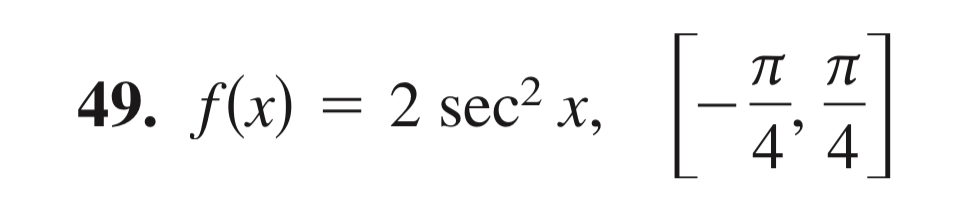Question
20 views

Find the value(s) of c guaranteed by the Mean Value Theorem for Integrals for the function over the given interval.help_outlineImage Transcriptionclose49. f(x) = 2 sec3x -π π 4' 4 fullscreen
check_circle

Step 1

Mean value theorem:

The statement of the mean value theorem says that, if a function f(x) is continuous and differentiable on the interval [a, b], then there exists a number c from the interval [a, b] that,

Step 2

Required conditions for the given function to apply mean value theorem:

The given function is f(x) = 2*sec2x on the interval [(–π/4), (π/4)].

• The function f(x) = 2*sec2x must be continuous on the interval [(–π/4), (π/4)].
• The function f(x) = 2*sec2x must be differentiable.

It is known that the function f(x) = 2*sec2x will be continuous and differentiable on the interval [(–π/4), (π/4)]. Therefore, the conditions of mean value theorem are true.

Thus, there exists a number c in the interval [(–π/4), (π/4)] with,

Step 3

Find the value of f’(c):

The value of f’(c) is obtained from ...

### Want to see the full answer?

See Solution

#### Want to see this answer and more?

Solutions are written by subject experts who are available 24/7. Questions are typically answered within 1 hour.*

See Solution
*Response times may vary by subject and question.
Tagged in

### Calculus